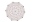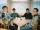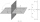# Quadratic equation + combinatorial number - math problems

#### Number of problems found: 8

• CombinationsIf the number of elements increase by 3, it increases the number of combinations of the second class of these elements 5 times. How many are the elements?
• DiagonalsWhat x-gon has 54 diagonals?
• CombinationsFrom how many elements we can create 990 combinations 2nd class without repeating?
• CombinationsHow many elements can form six times more combinations fourth class than combination of the second class?
• 2nd class combinationsFrom how many elements you can create 4560 combinations of the second class?
• VCP equationSolve the following equation with variations, combinations and permutations: 4 V(2,x)-3 C(2,x+ 1) - x P(2) = 0
• PartyAt the party everyone clink with everyone. Together, they clink 406 times. How many people were at the party?
• Points in spaceThere are n points, of which no three lie on one line and no four lies on one plane. How many planes can be guided by these points? How many planes are there if there are five times more than the given points?

We apologize, but in this category are not a lot of examples.
Do you have an interesting mathematical word problem that you can't solve it? Submit a math problem, and we can try to solve it.

We will send a solution to your e-mail address. Solved examples are also published here. Please enter the e-mail correctly and check whether you don't have a full mailbox.

Please do not submit problems from current active competitions such as Mathematical Olympiad, correspondence seminars etc...

Looking for help with calculating roots of a quadratic equation? See also our combinations calculator. Quadratic Equations Problems. Combinatorial number - math word problems.